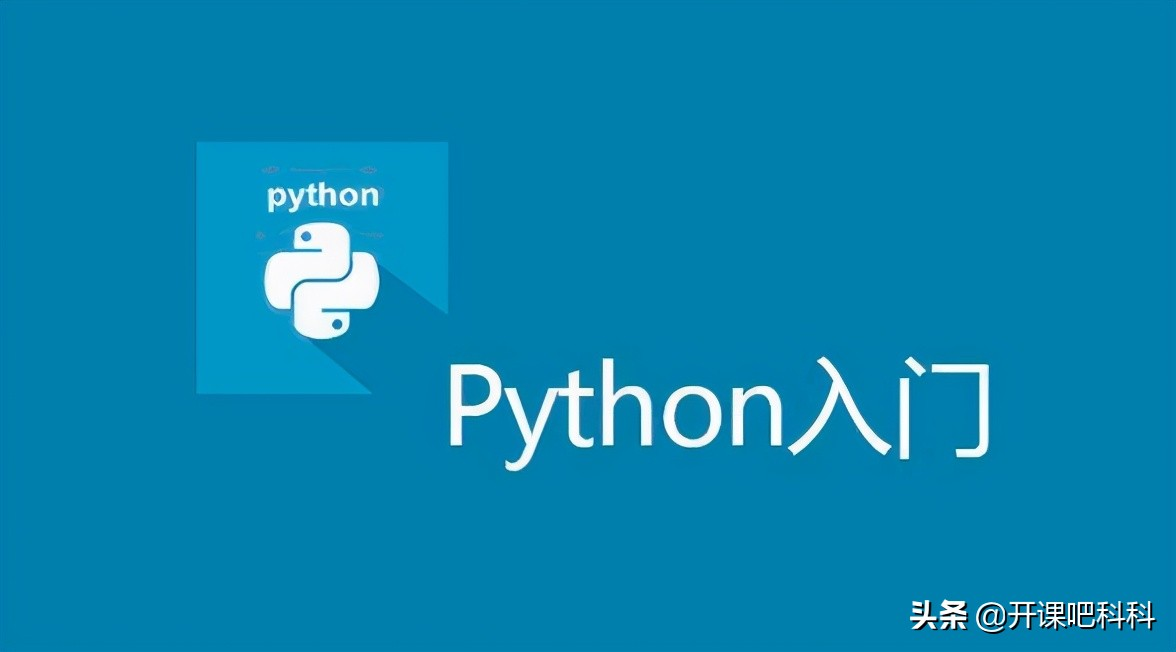# Python中的条件选择和循环语句

2021-07-14    开课吧科科# 一、条件选择语句

Python中条件选择语句的关键字为：if、elif、else这三个。其基本形式如下：

``````if condition:
block elif condition:
block
... else: block1234复制代码类型：[python]``````

``score=input() if score<60: print "D" elif score<80: print "C" elif score<90: print "B" else: print "A"1复制代码类型：[python]``

# 二、循环语句

for循环的基本形式如下：

``````for variable in list:
block12复制代码类型：[python]``````

``````sum=0 for var in range(1,101):
sum+=var print sum12复制代码类型：[python]``````

range()是一个内置函数，它可以生成某个范围内的数字列表。比如说range(1,6)就会生成[1,2,3,4,5]这样一个列表，而range(8)会生成[0,1,2,3,4,5,6,7]这样一个列表。

``````list=['China','England','America'] for i in range(len(list)):
word=list[i] for j in range(len(word)): print word[j]12复制代码类型：[python]``````

``````while condition:
block12复制代码类型：[python]``````

``count=2 while count>0: print "i love python!" count=count-11复制代码类型：[python]``

``count=5 while True: print "i love python!" count=count-1 if count==2: break1复制代码类型：[python]``
``````count=5 while count>0:
count=count-1 if count==3: continue print "i love python!" 12复制代码类型：[python]``````

``#这两段循环功能完全相同 for i in range(0,10): print i else: print 'over' for i in range(0,10): print i print 'over'1复制代码类型：[python]``

``#这两段循环功能完全相同  count=5 while count>0: print 'i love python' count=count-1 else: print 'over' count=5 while count>0: print 'i love python' count=count-1 print 'over'1复制代码类型：[python]``

▍相关推荐